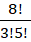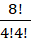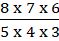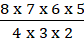# CAT 2019 Question Paper | Quants Slot 1

###### CAT Previous Year Paper | CAT Quants Questions | Question 3

This is probably amongst the tougher questions in the CAT 2019 question paper. It uses a variety of concepts including coordinate geometry and arrangements. All CAT previous year paper have these kind of questions that are extremely tough. While the CAT syllabus will probably classify this under arrangements, only practice will help you identify that there are multiple layers to the problem. Identifying these questions can come only with practice that you do as a part of your online CAT Preparation

Question 3 : With rectangular axes of coordinates, the number of paths from (1,1) to (8,10) via (4,6), where each step from any point (x,y) is either to (x,y+1) or to (x+1,y) is [TITA]

## Best CAT Coaching in Chennai

#### CAT Coaching in Chennai - CAT 2022Limited Seats Available - Register Now!

Let us first consider travelling from (1,1) to (4, 6)
This means, Travelling from 1 to 4 units in the x axis -> 3 horizontal movements (h h h)
And travelling from 1 to 6 units in the y axis -> 5 vertical movements (v v v v v)
No matter how we proceed, reaching from (1,1) to (4,6) requires 5 vertical movements and 3 horizontal movements.
So, Number of paths to travel from (1,1) to (4,6) = Number of ways of arranging (h h h v v v v v)
Number of ways of arranging (h h h v v v v v) =Similarly, travelling from (4, 6) to (8, 10) requires 4 horizontal movements and 4 vertical movements
Number of ways of arranging (h h h h v v v v) =Total number of paths =x=x= 3920

The question is "With rectangular axes of coordinates, the number of paths from (1,1) to (8,10) via (4,6), where each step from any point (x,y) is either to (x,y+1) or to (x+1,y) is [TITA] "

##### Hence, the answer is 3920

###### CAT Coaching in ChennaiCAT 2023

Classroom Batches Starting Now! @Gopalapuram

###### Best CAT Coaching in Chennai Introductory offer of 5000/-

Attend a Demo Class

##### Where is 2IIM located?

2IIM Online CAT Coaching
A Fermat Education Initiative,
58/16, Indira Gandhi Street,
Kaveri Rangan Nagar, Saligramam, Chennai 600 093

##### How to reach 2IIM?

Mobile: (91) 99626 48484 / 94459 38484
WhatsApp: WhatsApp Now
Email: info@2iim.com